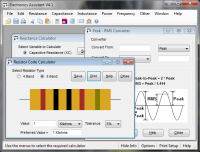The first two calculators below calculate series or parallel combinations of resistors. Enter the resistor value and press 'Add to Total'. Repeat until all resistors have been entered. Press 'Clear Total' to start a new calculation. The third calculator will find a resistor value to place in parallel with a given resistor value to produce a specified total. Preferred resistor values are also calculated, use the radio buttons on each calculator to select the preferred value series to use.

#### Resistors in Series - Calculate Total:

 Enter resistance, press 'Add to Total', repeat. Press 'Clear Total' to reset. Ohms Kilohms Megohms Select preferred series (optional): E12 E24 E48 E96 E192 Total R =? Preferred Total R =?

#### Resistors in Parallel - Calculate Total:

 Enter resistance, press 'Add to Total', repeat. Press 'Clear Total' to reset. Ohms Kilohms Megohms Select preferred series (optional): E12 E24 E48 E96 E192 Total R =? Preferred Total R =?

#### 2 Resistors in Parallel - Calculate One Resistor from Other and Total:

 Enter R1 and the total resistance to calculate the value of the other resistor needed to produce the required total. R1: Ohms Kilohms Megohms Total R: Ohms Kilohms Megohms Select preferred series (optional): E12 E24 E48 E96 E192 R2 = ? Preferred Total R = ?

#### Formulae

Resistors in series:

RTOTAL = R1 + R2 + R3 + ...

Resistors in parallel:

1 / RTOTAL = (1 / R1) + (1 / R2) + (1 / R3) + ...

See the technical data section for more details.

# If you found this calulator useful you should try Electronics Assistant!• All the online calculators and more in a stand-alone application
• Converts Resistor & Inductor colour codes, calculates LED series resistors, capacitance units, series / parallel resistors & capacitors, frequency, reactance & more
• Calculation of nearest preferred resistor values with a choice of 5 series from E12 to E192
• Print & save calculation results